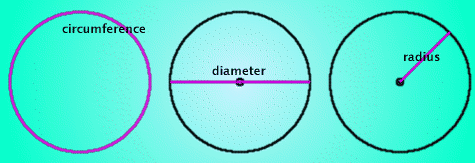# Pi, a Very Special Number

##### Age 7 to 14

Published 2011 Revised 2012

$\pi$ is the symbol used for a special number which we call pi (pronounced "pie"). $\pi$ comes from working with circles.#### $\pi$ is the ratio of the circumference of a circle to its diameter.

This means that you can work out $\pi$ by dividing the distance around a circle by the length of its diameter. The strange thing is that on a calculator the answer you get can only ever be approximate - that is, you can't show an exact value for pi with digits on a calculator screen or even on large pieces of paper. This has fascinated mathematicians for a very long time and they have kept trying to find ways to calculate approximations of pi that are more and more accurate.

#### $\pi$ is needed to find the area of a circle using the formula $\pi{r}^{2}$

To work out the area of a circle you need to use an approximate value for pi (like $3.14$) then multiply that by the radius of the circle squared. For example; if the radius was $5 \; \text{cm}$, the area would be approximately $3.14 \times 5 \times 5 \times 78.5$ square centimetres.

What is the value of Pi?

In the Old Testament of the Bible (I Kings 7:23) it is suggested that $\pi$ is equal to $3$. The Babylonians, in about 2000 BC, use $3$ or $3 1/8$. In the ancient Rhind papyrus, the Egyptian scribe Ahmes said that $\pi$ was equal to $16/9$ squared. This calculates to $3.16049 \ldots$.

Archimedes worked on the problem of finding $\pi$ by calculating the area of regular polygons, with up to $96$ sides. He decided that $\pi$ was somewhere between $310/71$ and $310/70$. In decimals this would be $3.14085 \ldots$ and $3.142857 \ldots$ (remember the decimal places keep going on and on).

Over the centuries, many mathematicians, such as Ptolemy (the ancient Greek astronomer), Tsu Ch'ung-Chi (of China) and Ludolph van Ceulen (of Germany) kept trying to find more accurate values for pi using a variety of different methods. During the last few centuries people have been trying to find as many decimal places as possible so they can look for patterns in the long string of digits. In 1949 a computer was used to calculate pi to $2\;037$ places. In 1967 in France $500\;000$ digits were found. In 1983 a Japanese team found $16\;777\;216$ decimal places for pi. The current record is about $51$ billion decimal places.

Here are some of the decimal places that have been found: $$\pi = 3.14159 \; 26535 \; 89793 \; 23846 \; 26433 \; 83279 \; 50288 \; 41972 \; \dots$$ To help remember these digits, people like to make up sentences or rhymes, called mnemonics.

For example, "May I have a large container of coffee?" is quite a famous one for the first eight digits. You work out the numbers by counting the letters in each word.

Here's one for the first 31 digits:

"Now I will a rhyme construct,
By chosen words the young instruct.
Cunningly devised endeavour,
Con it and remember ever.
Widths in circle here you see,
Sketched out in strange obscurity."

Try making up your own mnemonic for the digits of pi.

To find out more about pi, have a look at Mathworld - although some of the topics it covers are very advanced!
http://mathworld.wolfram.com/Pi.html